GeeksforGeeks App
Open AppBrowser
Continue

## Related Articles

There are some filters that are added in the AngularJS for the sake of making the formatting and working with data easier. There are several in-built filters in AngularJS. They are listed here along with some examples to make the understanding easier.

Basic Syntax:
Filters are generally added to the expressions by using the pipe (|) character.
For example, the filter {{ fullName | uppercase }} formats the fullName into the uppercase format.

Some of the pre-built filters in AngularJS are:

• currency The number is formatted to currency format.
• date The date is specified to a specific format.
• filter The array is filtered on the basis of the provided criteria.
• limitTo The array or an string is limited into a specified number of elements/characters.
• number A number if formatted to a string.
• orderBy The array is ordered by an expression.
• lowercase This filter converts a string to lowercase letters.
• uppercase This filter converts a string to uppercase letters.
• json It converts a JavaScript object into a JSON string.

1. Currency Filter:
This filter simply formats a number as currency.

 ```<``html``>``<``script` `src``=``"https://ajax.googleapis.com/ajax/libs/angularjs/1.6.9/angular.min.js"``>``  ```` ` `<``body``>`` ` `    ``<``div` `ng-app``=``"myApp"` `ng-controller``=``"costCtrl"``>`` ` `        ``<``h1``>Currency Format - GeeksforGeeks``        ``<``h2``>Price: {{ price | currency }}`` ` `    ```` ` `    ``<``script``>``        ``var app = angular.module('myApp', []);``        ``app.controller('costCtrl', function(\$scope) {``            ``\$scope.price = 20;``        ``});``    ```` ` ``` ` ``

Output: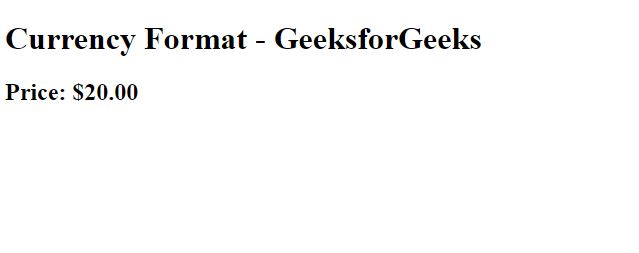Here, the dollar (\$) sign has been added automatically in front of the numerical value.

2. Date Filter:
The date filter formats a date to a specified format.

Syntax:

`{{ date | date : format : timezone }}`

 ```<``html``>``<``script` `src``=``"https://ajax.googleapis.com/ajax/libs/angularjs/1.6.9/angular.min.js"``>``  ```` ` `<``body``>`` ` `    ``<``div` `ng-app``=``"myApp"` `ng-controller``=``"datCtrl"``>`` ` `        ``<``h1``>GeeksforGeeks - Date Filter``        ``<``p``>Date = {{ today | date }}`` ` `    ```` ` `    ``<``script``>``        ``var app = angular.module('myApp', []);``        ``app.controller('datCtrl', function(\$scope) {``            ``\$scope.today = new Date();``        ``});``    ```` ` ``` ` ``

Output: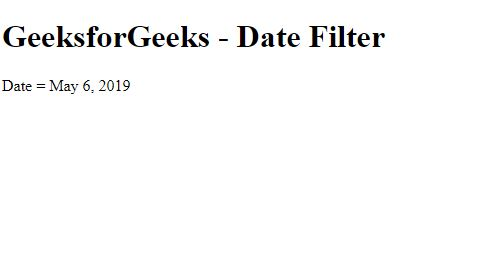Here, the date() function has been used and that displays the current date.

3. Filter:
This is used to display only the required objects. The filter selects a subset of an array.
For example, This filter can be used only on arrays as this returns an array containing only the matching items(condition given in the array).

 ```<``html``>``<``script` `src``=``"https://ajax.googleapis.com/ajax/libs/angularjs/1.6.9/angular.min.js"``>``  ```` ` `<``body``>`` ` `    ``<``div` `ng-app``=``"myApp"` `ng-controller``=``"namesCtrl"``>`` ` `        ``<``h1``>filter - GeeksforGeeks`` ` `        ``<``ul``>``            ``<``li` `ng-repeat``=``"x in names | filter : 'e'"``>``                ``{{ x }}``            ````        ```` ` `    ```` ` `    ``<``script``>``        ``angular.module('myApp', []).controller('namesCtrl',``                                               ``function(\$scope) {``            ``\$scope.names = [``                ``'Jani',``                ``'Carl',``                ``'Margareth',``                ``'Hege',``                ``'Joe',``                ``'Gustav',``                ``'Birgit',``                ``'Mary',``                ``'Kai'``            ``];``        ``});``    ```` ` `    ``<``p``>This example displays only the names ``      ``containing the letter "e".`` ` ``` ` ``

Output: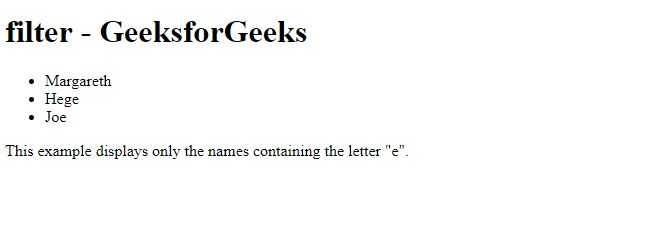4. limitTo Filter:
This filter returns an array or a string containing only a specified number of elements. The output will depend on the type of input that is given to the program. When used for arrays, it returns an array containing only the specified number of items.
In the case of the string, it returns a string containing, only the specified number of characters, while when used for numbers, it returns a string containing only the specified number of digits.

Syntax:

`{{ object | limitTo : limit : begin }}`

Here, limit specifies the number of elements to be displayed, while begin specifies where to begin the limitation from.

 ```<``html``>``<``script` `src``=``"https://ajax.googleapis.com/ajax/libs/angularjs/1.6.9/angular.min.js"``>``  ```` ` `<``body``>`` ` `    ``<``div` `ng-app``=``"myApp"` `ng-controller``=``"sizeCtrl"``>`` ` `        ``<``h1``>limitTo - GeeksforGeeks``        ``<``ul``>``            ``<``li` `ng-repeat``=``"x in cars | limitTo : 4 : 1"``>{{x}}``        ```` ` `    ```` ` `    ``<``script``>``        ``var app = angular.module('myApp', []);``        ``app.controller('sizeCtrl', function(\$scope) {``            ``\$scope.cars = ["Audi",``                           ``"BMW", ``                           ``"Dodge", ``                           ``"Fiat",``                           ``"Ford",``                           ``"Volvo", ``                           ``"Lamborghini"];``        ``});``    ```` ` `    ``<``p``>Filter applied from first ``      ``element to the fifth element.`` ` ``` ` ``

Output: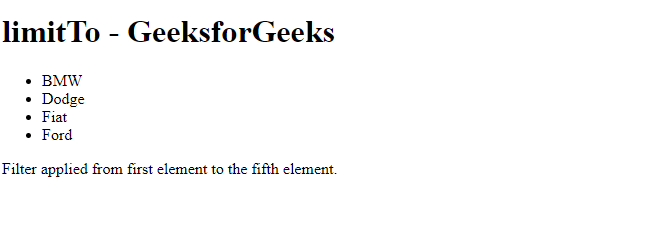5. orderBy Filter:
This is used for sorting an array. Strings (default alphabetically) and numbers (default ascending) can be sorted using this filter.

Syntax:

`{{ array | orderBy : expression : reverse }}`

Here, the reverse can be used to reverse the order of the resulting array.

 ```<``html``>``<``script` `src``=``"https://ajax.googleapis.com/ajax/libs/angularjs/1.6.9/angular.min.js"``>``  ```` ` `<``body``>`` ` `    ``<``div` `ng-app``=``"myApp"` `ng-controller``=``"orderCtrl"``>`` ` `        ``<``h1``>orderBy - GeeksforGeeks``        ``<``ul``>``            ``<``li` `ng-repeat``=``"x in customers | orderBy : 'city'"``>``                ``{{x.name + ", " + x.city}}``            ````        ```` ` `    ```` ` `    ``<``script``>``        ``var app = angular.module('myApp', []);``        ``app.controller('orderCtrl', function(\$scope) {``            ``\$scope.customers = [{``                ``"name": "Delhi"``            ``}, {``                ``"name": "Mumbai"``            ``}, {``                ``"name": "Patna"``            ``}, {``                ``"name": "Kolkata"``            ``}, {``                ``"name": "Pune"``            ``}, {``                ``"name": "Ranchi"``            ``}, {``                ``"name": "Bhopal"``            ``}];``        ``});``    ```` ` `    ``<``p``>Sorting in ascending order.`` ` ``` ` ``

Output: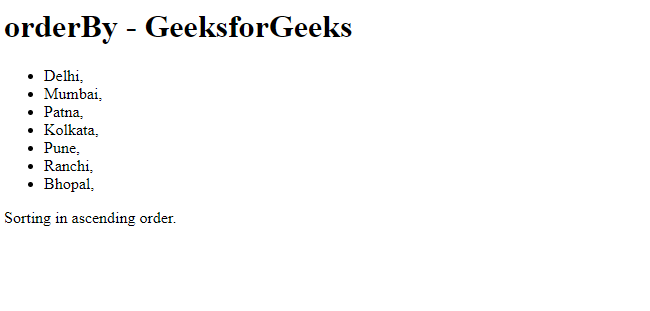6. number Filter:
Probably the simplest filter. It simply formats a number to a string.

Syntax:

`{{ string | number : fractionsize}}`

Here, ‘fractionsize’ specifies the number of decimals.

 ```<``html``>``<``script` `src``=``"https://ajax.googleapis.com/ajax/libs/angularjs/1.6.9/angular.min.js"``>`` ````<``body``>`` ` `<``div` `ng-app``=``"myApp"` `ng-controller``=``"nCtrl"``>`` ` `<``h1``>number Filter - GeeksforGeeks``<``h2``>Rs.{{money | number : 3}}`` ` ``` ` `<``script``>``var app = angular.module('myApp', []);``app.controller('nCtrl', function(\$scope) {``    ``\$scope.money = 999999;``});```` ` `<``p``>The money is written with three decimals.`` ` ````

Output: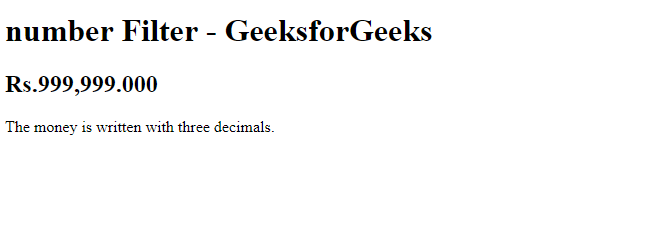7. lowercase Filter:
This filter simply converts a string to lowercase letters.

Syntax:

`{{ string | lowercase }}`

 ```<``html``>``<``script` `src``=``"https://ajax.googleapis.com/ajax/libs/angularjs/1.6.9/angular.min.js"``>```` ` `<``body``>`` ` `    ``<``h2``>AngularJS - lowercase``    ``<``br``>``    ``<``br``>`` ` `    ``<``div` `ng-app``=``"myApp"` `ng-controller``=``"myCtrl"``>`` ` `        ``<``strong``>Input:``        ``<``br``>``        ``<``input` `type``=``"text"` `ng-model``=``"string"``>``        ``<``br``>``        ``<``br``>``        ``<``strong``>Output:``        ``<``br``> {{string|lowercase}}`` ` `    ```` ` `    ``<``script``>``        ``var app = angular.module('myApp', []);``        ``app.controller('myCtrl', function(\$scope) {``            ``\$scope.string = "";``        ``});``    ```` ` ``` ` ``

The code mentioned above asks the user for an input. After the user enters a term in the input box, it gets stored in the ng-model=”string”. Now, the AngularJS will resolve the expression, and return the result exactly where the expression is written. AngularJS expressions can be written inside double braces, like this: {{ expression }}.
Output: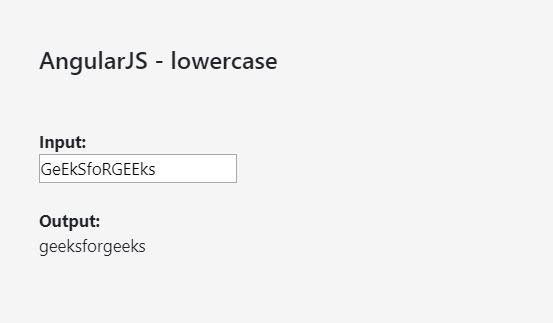Here in this code, the output {{string}} is displayed just below the input box. However, to change the input string to lowercase, ‘|lowercase’ must be added to the expression’s name.

Therefore, {{string|lowercase}} will return the input string in the lowercase format.

8. uppercase Filter:
The uppercase Filter in AngularJS is used to change a string to uppercase string or letters.

Syntax:

`{{ string | uppercase}}`

 ```<``html``>``<``script` `src``=``"https://ajax.googleapis.com/ajax/libs/angularjs/1.6.9/angular.min.js"``>````<``body``>`` ` `<``div` `ng-app``=``"myApp"` `ng-controller``=``"caseCtrl"``>`` ` `<``h1``>{{txt | uppercase}}`` ` ``` ` `<``script``>``var app = angular.module('myApp', []);``app.controller('caseCtrl', function(\$scope) {``    ``\$scope.txt = "GeeksforGeeks!";``});```` ` `<``p``>The text is written in uppercase letters.`` ` ````

Output: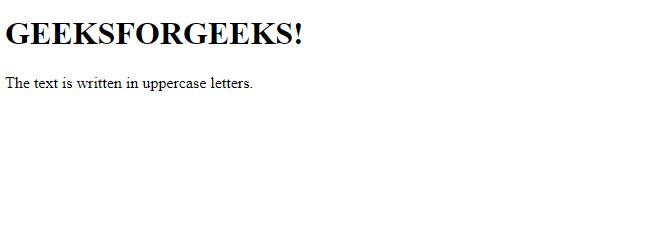9. json Filter:
This filter simply converts a JavaScript object into a JSON string, and this is very much useful while the debugging of applications.

Syntax:

`{{ object | json : spacing }}`

Here, spacing specifies the number of spaces to use per indentation. The default value is 2, however, this value is optional.

Have a look at this example code:

 ```<``html``>``<``script` `src``=``"https://ajax.googleapis.com/ajax/libs/angularjs/1.6.9/angular.min.js"``>````<``body``>`` ` `<``div` `ng-app``=``"myApp"` `ng-controller``=``"jsCtrl"``>`` ` `<``h1``>GeeksforGeeks`` ` `<``pre``>{{customer | json : 20}}`` ` ``` ` `<``script``>``var app = angular.module('myApp', []);``app.controller('jsCtrl', function(\$scope) {``    ``\$scope.customer = {``        ``"name" : "Milk",``        ``"city" : "Patna",``        ``"country" : "India"``    ``};``});```` ` `<``p``>A JSON string with 20 spaces per indentation.`` ` ````

Output: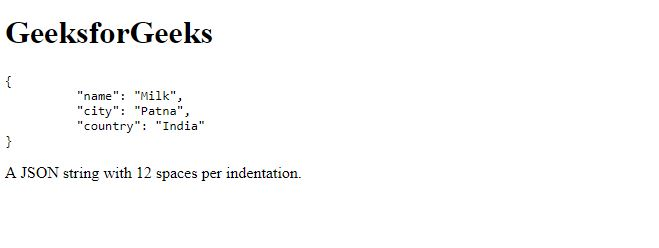10. Let’s have a look at the example of an array filter.

 ```<``html``>`` ` `<``body``>`` ` `    ``<``h1``>GeeksforGeeks`` ` `    ``<``p``>Click the button to get every element``      ``in the array that has a value of 38 or more.`` ` `    ``<``button` `onclick``=``"myFunction()"``>Try it`` ` `    ``<``p` `id``=``"demo"``>`` ` `    ``<``script``>``        ``var num = [23, 32, 56, 30, 56, 45, 34, 39];`` ` `        ``function checkNum(num) {``            ``return num >= 38;``        ``}`` ` `        ``function myFunction() {``            ``document.getElementById(``                ``"demo").innerHTML = num.filter(checkNum);``        ``}``    ```` ` ``` ` ``

On clicking the ‘Try it’ button, all the elements in the array that has a value of 38 or more gets printed to the screen.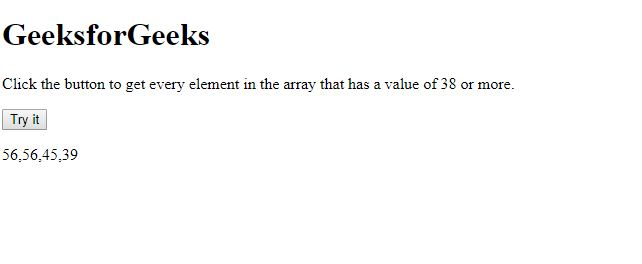My Personal Notes arrow_drop_up
Related Tutorials